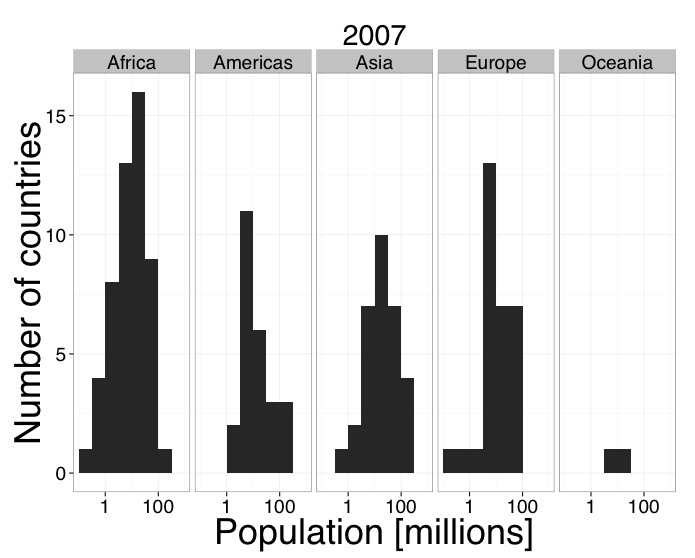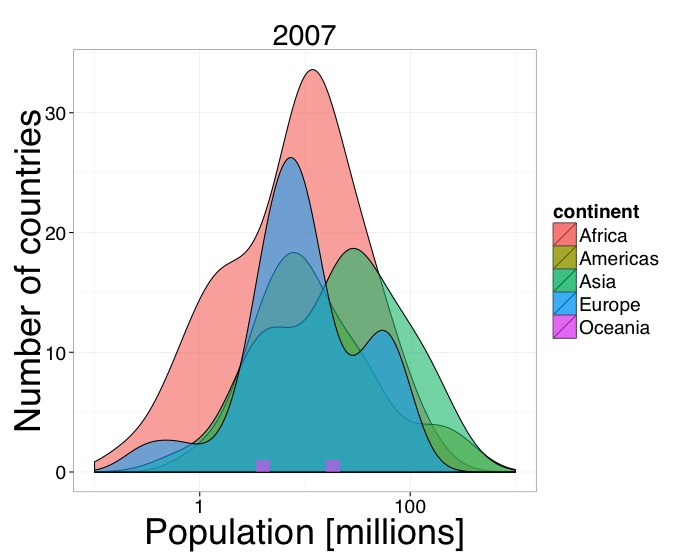# Faceted Table of Histograms

## Overview

Teaching: 5 min
Exercises: 15 min
Questions
• What kind of scientific question does a faceted table of charts address?

• How do I generate a faceted table with `ggplot2`?

Objectives
• To generate a faceted table of histograms using `ggplot2`

Build student independence to walk through decision making steps from question to chart.

• “How was the world population distributed among continents in 2007?”
• Comparison among many items or categories
• Faceted table of histogram bar charts

Implement faceted table of histograms.

• `facet_grid()`
• `lims()`

## Fix the Faceted Table Code Challenge

Fix the code to generate a faceted table of histograms.

``````ggplot(pop_2007, aes(year)) +
geom_histogram(binwidth=0.5) +
scale_x_log10(limits = c(0.1, 10))
facet_grid(continent ~ .) +
labs(x = Population [millions], y = Number of countries,
title = "2007") +
theme_bw(base_size = 42, base_family = "Script") +
theme(axis.title=element_text(size=36))
``````

## Output Solution## Code Solution

``````ggplot(pop_2007, aes(population/1000000)) +             # Replace aes()
geom_histogram(binwidth=0.5) +
scale_x_log10(limits = c(0.1, 1000)) +                 # Add +
facet_grid(. ~ continent) +                            # Reverse ~ .
labs(x = "Population [millions]", y = "Number of countries",
title = "2007") +                                 # Add ""
theme_bw(base_size = 24, base_family = "Helvetica") +  # Fix input vars
theme(axis.title = element_text(size = 36))
``````

## Alternate Good Charts Discussion

This is another quality solution to addressing “How was the world population distributed among continents in 2007?”

Compare and contrast the two graphic representations addressing the research question.## Grouped Density Chart Code

``````ggplot(pop_2007, aes(x = population/1000000, y = ..count.., fill = continent)) +
geom_density(alpha = 0.6) +
geom_histogram(data=filter(pop_2007, continent=="Oceania"), alpha = 0.6) +
scale_x_log10(limits = c(0.1, 1000)) +
labs(x = "Population [millions]", y = "Number of countries", title = "2007") +
theme_bw(base_size = 24, base_family = "Helvetica") +
theme(axis.title = element_text(size = 36))
``````

## Key Points

• Faceted tables represent a comparison among many items or categories.

• Multiple good charts can address a single scientific question.